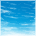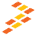# RTOS independant ISR

cancel
Showing results for
Show  only  | Search instead for
Did you mean:

## RTOS independant ISR

1,129 ViewsContributor III

I need to have a direct interrupt, which means MQX RTOS must not drive this interrupt. These interrupts come from Input Capture on all DMA and GPT pins. I managed to solve the problem using codewarrior 7.1 and MQX 3.3 and the following code. Unfortunately this is not working on Codewarrior 7.2 and MQX 3.61. What could I be missing? or what changes on MQX and compiler might affect the behavior of a working code?

`extern void main_task(uint_32);const TASK_TEMPLATE_STRUCT  MQX_template_list[] = {    /* Task Index,   Function,   Stack,  Priority, Name,     Attributes,          Param, Time Slice */    { MAIN_TASK,   main_task, 1500,   5,        "main",  MQX_AUTO_START_TASK, 0,     0 },    { 0 }};__declspec(interrupt:0) void DMA_T3_ISR(pointer dummy_param){   VMCF5225_STRUCT_PTR reg_ptr = (VMCF5225_STRUCT_PTR)BSP_IPSBAR;      reg_ptr->DMA_TIMER[ 3 ].DTER =  0b00000011; //Apaga las Banderas de Interrupcion}__declspec(interrupt:0) void DMA_T2_ISR(pointer dummy_param){   VMCF5225_STRUCT_PTR reg_ptr = (VMCF5225_STRUCT_PTR)BSP_IPSBAR;      reg_ptr->DMA_TIMER[ 2 ].DTER =  0b00000011; //Apaga las Banderas de Interrupcion}/*Prueba para lector y tarjeta juntos*/__declspec(interrupt:0) void DMA_T1_ISR(pointer dummy_param){   VMCF5225_STRUCT_PTR reg_ptr = (VMCF5225_STRUCT_PTR)BSP_IPSBAR;      reg_ptr->DMA_TIMER[ 1 ].DTER =  0b00000011; //Apaga las Banderas de Interrupcion}__declspec(interrupt:0) void DMA_T0_ISR(pointer dummy_param){   VMCF5225_STRUCT_PTR reg_ptr = (VMCF5225_STRUCT_PTR)BSP_IPSBAR;      reg_ptr->DMA_TIMER[ 0 ].DTER =  0b00000011; //Apaga las Banderas de Interrupcion}void main_task   (      uint_32 initial_data   ){VMCF5225_STRUCT_PTR reg_ptr = (VMCF5225_STRUCT_PTR)BSP_IPSBAR;     reg_ptr->GPIO.PORTTC = 0x00;  /*GPIO Configuration for DMA Edge Triggered Interrupt 0 = Output low level*/     reg_ptr->GPIO.DDRTC = 0x00;   /*GPIO Configuration for DMA Edge Triggered Interrupt 0 = Direction Input*/     reg_ptr->GPIO.PORTTCP_SETTC = 0x00;  /*GPIO Configuration for DMA Edge Triggered Interrupt Nothing since it is input*/     reg_ptr->GPIO.CLRTC = 0x00;   /*GPIO Configuration for DMA Edge Triggered Interrupt Nothing since it is input*/     reg_ptr->GPIO.PTCPAR = 0x55;  /*GPIO Configuration for DMA Edge Triggered Interrupt Primary Function for all DTIM pins*/  reg_ptr->ICTRL0.ICR = 0b01110011;  //DES DMA_Timer set to IL=7, IP=3     reg_ptr->ICTRL0.ICR = 0b01110010;  //DES DMA_Timer set to IL=7, IP=2     reg_ptr->ICTRL0.ICR = 0b01110001;  //DES DMA_Timer set to IL=7, IP=1  reg_ptr->ICTRL0.ICR = 0b01110000;  //DES DMA_Timer set to IL=7, IP=0    reg_ptr->DMA_TIMER[ 0 ].DTMR =  0b0000000010000011;   reg_ptr->DMA_TIMER[ 1 ].DTMR =  0b0000000010000011;   reg_ptr->DMA_TIMER[ 2 ].DTMR =  0b0000000010000011;      reg_ptr->DMA_TIMER[ 3 ].DTMR =  0b0000000010000011;           reg_ptr->DMA_TIMER[ 0 ].DTXTMR = 0b01000000;//Everything Zero in order to:     reg_ptr->DMA_TIMER[ 1 ].DTXTMR = 0b01000000;//1.- Have the timer increment by one     reg_ptr->DMA_TIMER[ 2 ].DTXTMR = 0b01000000;//2.- Interrupt Enabled and DMA request disabled     reg_ptr->DMA_TIMER[ 3 ].DTXTMR = 0b01000000;//3.- Timer Continues if core halts ( Dont need this feature , just trying to see if it works like this)     reg_ptr->DMA_TIMER[ 0 ].DTER =  0b00000011;  //Clear event condition     reg_ptr->DMA_TIMER[ 1 ].DTER =  0b00000011;  //Clear event condition     reg_ptr->DMA_TIMER[ 2 ].DTER =  0b00000011;  //Clear event condition     reg_ptr->DMA_TIMER[ 3 ].DTER =  0b00000011;  //Clear event condition     reg_ptr->DMA_TIMER[ 0 ].DTCN =  0x0000;       reg_ptr->DMA_TIMER[ 1 ].DTCN =  0x0000;      reg_ptr->DMA_TIMER[ 2 ].DTCN =  0x0000;      reg_ptr->DMA_TIMER[ 3 ].DTCN =  0x0000;      *(unsigned int *) 0x2000014C = (unsigned int)&DMA_T0_ISR; //Install direct ISR vector for DTIM3     *(unsigned int *) 0x20000150 = (unsigned int)&DMA_T1_ISR; //Install direct ISR vector for DTIM3     *(unsigned int *) 0x20000154 = (unsigned int)&DMA_T2_ISR; //Install direct ISR vector for DTIM3  *(unsigned int *) 0x20000158 = (unsigned int)&DMA_T3_ISR; //Install direct ISR vector for DTIM3     _interrupt_controller_unmask(MCF5225_INT_DTIM0); //DES unmask DTIM3     _interrupt_controller_unmask(MCF5225_INT_DTIM1); //DES unmask DTIM3     _interrupt_controller_unmask(MCF5225_INT_DTIM2); //DES unmask DTIM3     _interrupt_controller_unmask(MCF5225_INT_DTIM3); //DES unmask DTIM3         while(1)   {       _time_delay(200);      }}`

3 Replies
327 ViewsContributor IV

Do yo get error in making the project or doesn't it work at all?

You can take a look at this....

Do you have MQX_ROM_VECTORS set to 1 in user_config.h?

Regards,

Marco

327 ViewsContributor III

The project builds and programs correctly but interrupts are never called.

I checked the thread you mention but I know the registers are correctly configured so that is not part of the problem.

I had  MQX_ROM_VECTORS = 0 but then I changed it to 1 and the problem continues.

327 ViewsNXP Employee

Check your vector table if it is correct. Then, check the interrupt controller settings (interrupt mask bit, interrupt mask all bit).